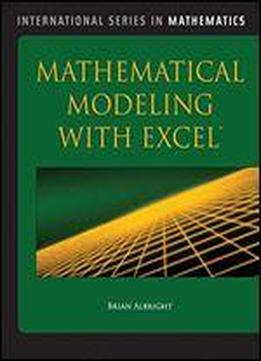# Mathematical Modeling With Excel by Brian Albright / 2009 / English / EPUB

Mathematical Modeling with Excel presents various methods used to build and analyze mathematical models in a format that students can quickly comprehend. Excel is used as a tool to accomplish this goal of building and analyzing the models. Ideal for math and secondary math education majors, this text presents a wide variety of common types of models, as well as some new types, and presents each in a unique, easy-to-understand format.

views: 223